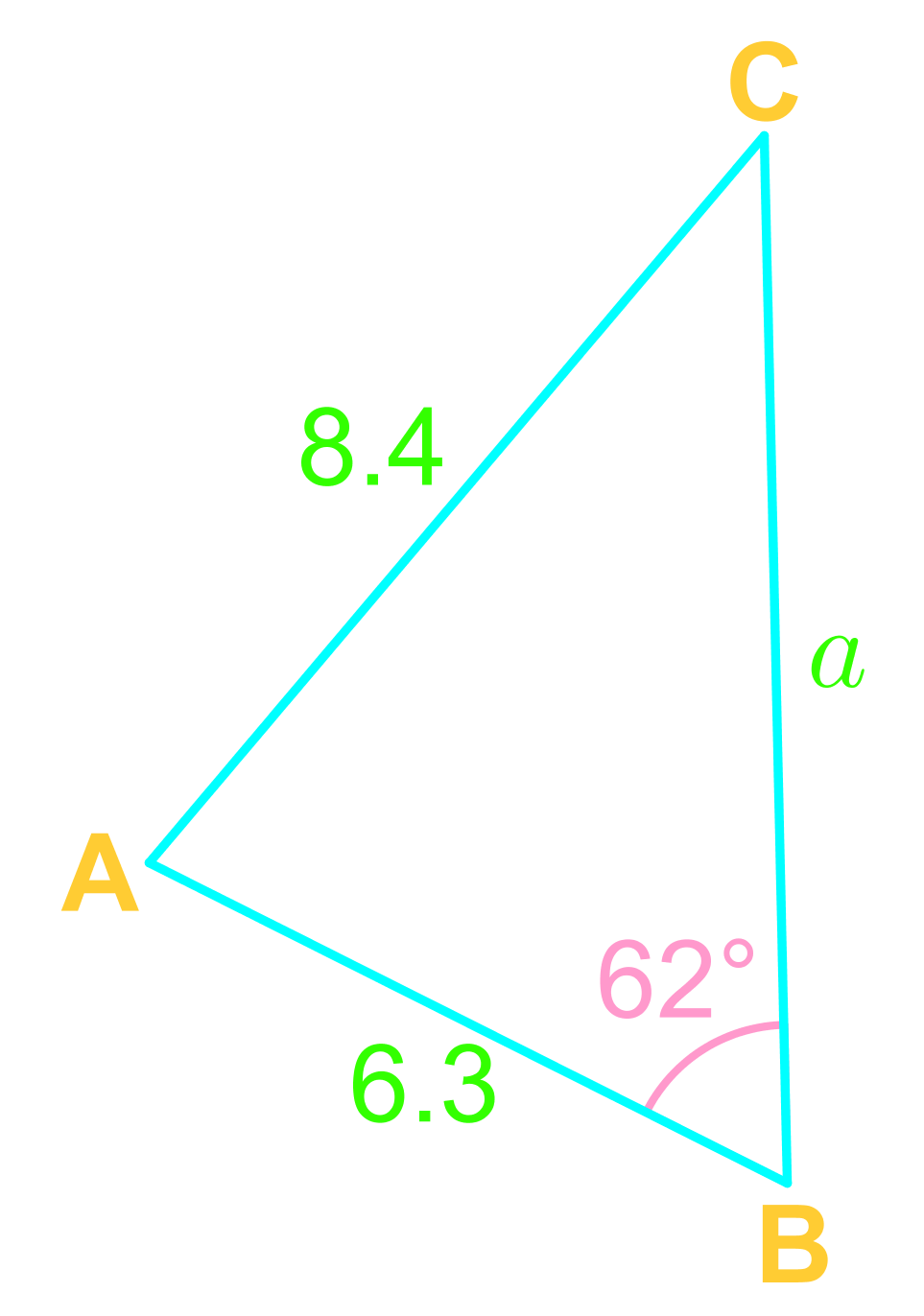Law of sines - Trigonometric Ratios and Angle Measures

Law of sines

In this section, we will learn about the Law of Sines, also known as the Sines Rule. The Law of Sines is a formula that models the relationship between the sides and the angles of any triangle, be it a right-angled triangle, an obtuse triangle, or an acute triangle. In order to use the Law of Sines, we need to satisfy the "one pair, one additional information" condition (i.e. Angle-Angle-Side abbreviated as AAS, and Angle-Side-Angle abbreviated as ASA). We will also explore the concept of the Ambiguous Case of the Law of Sines.

Lessons

Given the following triangle $\triangle ABC$,